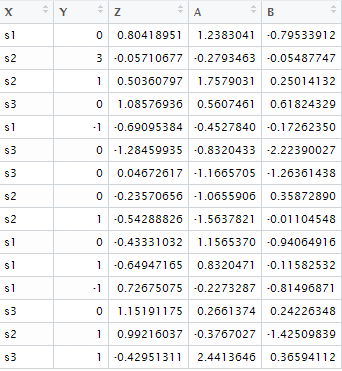# R : Summarize Data

This tutorial explains how to aggregate or summarize data.

Create a sample data
set.seed(1)
data <- data.frame(X = paste("s", sample(1:3, 15, replace = TRUE), sep = ""),Y = ceiling(rnorm(15)), Z = rnorm(15), A = rnorm(15), B = rnorm(15))Sample Data
Calculate Mean of Z by grouping variable X
dat1 = aggregate(Z ~ X, data=data, FUN=mean)
Calculate Mean of Z by 2 grouping variables
dat2 = aggregate(Z~ X + Y, data=data, FUN=mean)
Calculate Mean of Y and Z by grouping variable X
dat3 = aggregate(cbind(Y,Z)~X, data=data, FUN=mean)
Calculate Mean of all the variable by grouping variable X
dat4 = aggregate(.~X, data=data, FUN=mean)

Concatenate Text Based on Criteria
testDF <- data.frame(v1 = c(1,3,5,7,8,3,5,NA,4,5,7,9),
v2 = c(11,33,55,77,88,33,55,25,44,55,77,99) )
aggregate(v2 ~ v1, data = testDF, FUN=paste, sep=",")Share
Related Posts1.